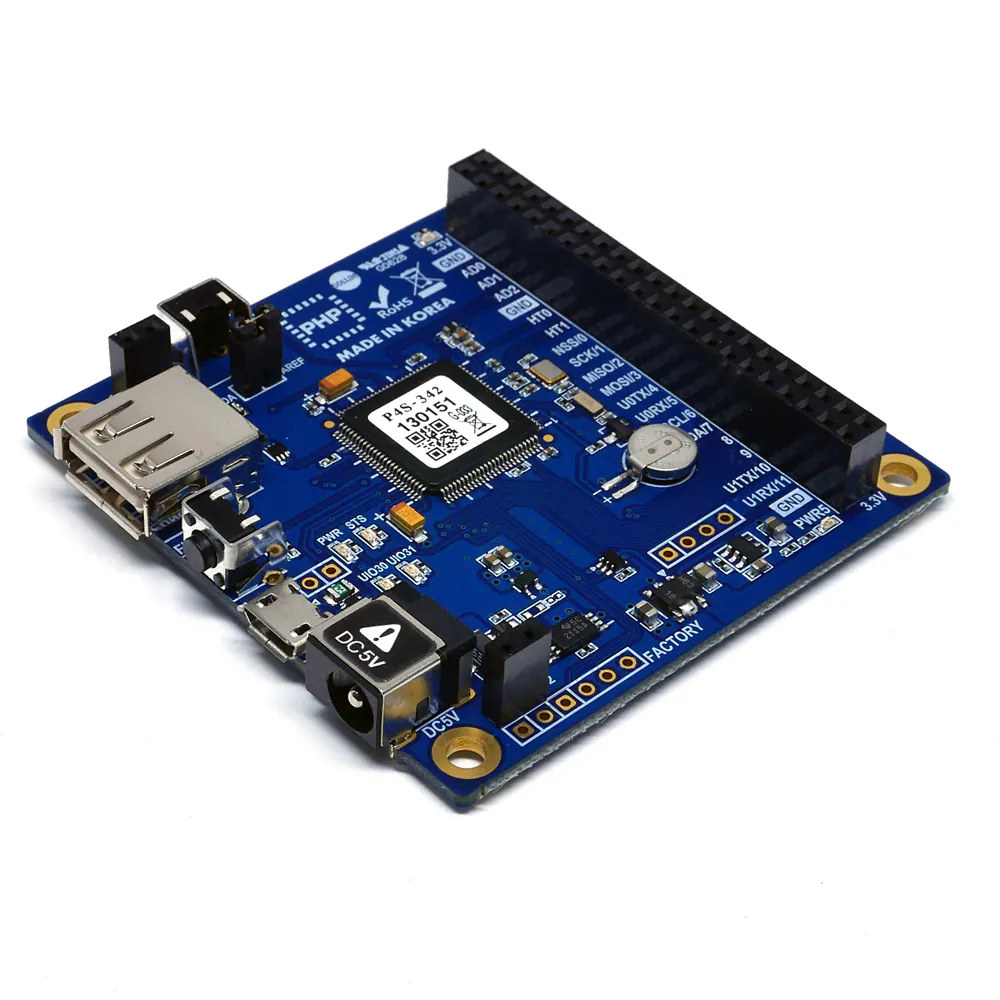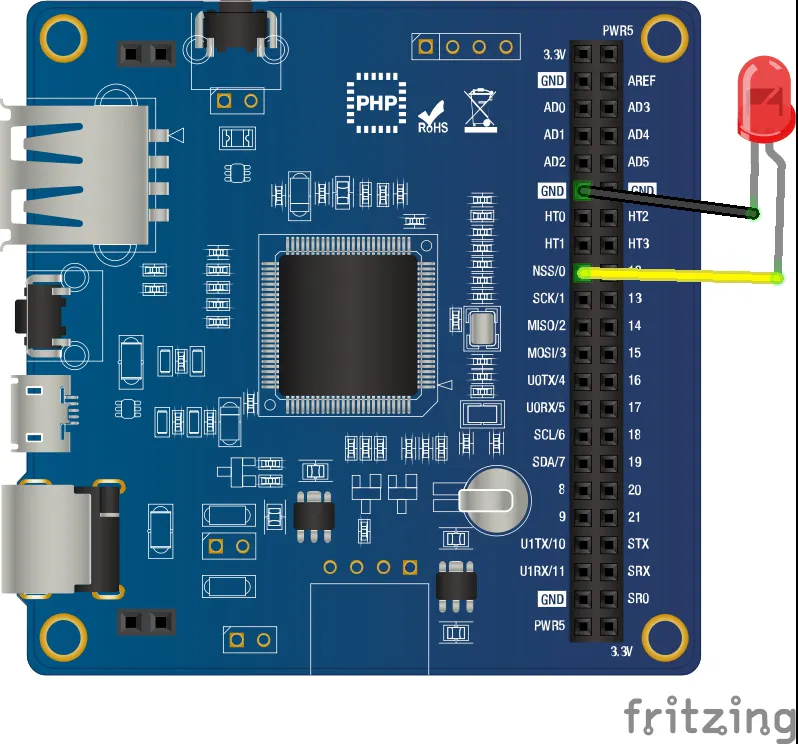# Web-Based Pattern Unlock for IoT device

This project prevents unauthorized people from controlling devices by using unlock pattern.

BeginnerFull instructions provided2,989

## Things used in this project

### Hardware componentsPHPoC Blue
×1
 LED
×1UTSOURCE Electronic Parts
×1

## Schematics

### wiring_rV5AOOQak2.jpg## Code

### User Interface (index.php)

PHP
```<!DOCTYPE html>
<html>
<title>Arduino - PHPoC Shield</title>
<meta name="viewport" content="width=device-width, initial-scale=0.7, maximum-scale=0.7">
<meta charset="utf-8">
<style>
body { text-align: center; font-size: width/2pt; }
h1 { font-weight: bold; font-size: width/2pt; }
h2 { font-weight: bold; font-size: width/2pt; }
button { font-weight: bold; font-size: width/2pt; }
</style>
<script>
var canvas_width = 400, canvas_height = 400;
var gap = 120;
var ws;
var touch_state = 0;
var touch_x = 0, touch_y = 0;
var touch_list = new Array();

var ratio = 1;

var authorized = false;
var light_state = 0;

function init()
{
var width = window.innerWidth;
var height = window.innerHeight;

if(width < height)
ratio = (width - 10) / canvas_width;
else
ratio = (height - 100) / canvas_width;

canvas_width = Math.round(canvas_width*ratio);
canvas_height = Math.round(canvas_height*ratio);

var canvas = document.getElementById("remote");
canvas.width = canvas_width;
canvas.height = canvas_height;

var ctx = canvas.getContext("2d");
ctx.translate(canvas_width/2, canvas_height/2);
ctx.lineCap="round";
ctx.lineJoin="round";

update_view();
}
function connect_onclick()
{
if(ws == null)
{
if((navigator.platform.indexOf("Win") != -1) && (ws_host_addr.charAt(0) == "["))
{
// network resource identifier to UNC path name conversion
}

ws = new WebSocket("ws://" + ws_host_addr + "/web_pattern", "text.phpoc");
document.getElementById("ws_state").innerHTML = "CONNECTING";
ws.onopen = ws_onopen;
ws.onclose = ws_onclose;
ws.onmessage = ws_onmessage;
}
else
ws.close();
}
function ws_onopen()
{
document.getElementById("ws_state").innerHTML = "<font color='blue'>CONNECTED</font>";
document.getElementById("bt_connect").innerHTML = "Disconnect";
update_view();
}
function ws_onclose()
{
document.getElementById("ws_state").innerHTML = "<font color='gray'>CLOSED</font>";
document.getElementById("bt_connect").innerHTML = "Connect";
ws.onopen = null;
ws.onclose = null;
ws.onmessage = null;
ws = null;
authorized = false;
update_view();
}
function ws_onmessage(e_msg)
{
e_msg = e_msg || window.event; // MessageEvent

var cmd = Number(e_msg.data);

if(cmd == 202)
authorized = true;
else if(cmd == 401)
authorized = false;

update_view();
}
function update_view()
{
var canvas = document.getElementById("remote");
var ctx = canvas.getContext("2d");

ctx.clearRect(-canvas_width/2, -canvas_height/2, canvas_width, canvas_height);

if(!authorized)
{
ctx.fillStyle = "black";
ctx.beginPath();
ctx.arc(0, 0, canvas_width/2, 0, 2 * Math.PI);
ctx.fill();

// draw touched point and line
ctx.lineWidth = 10;
ctx.strokeStyle="white";
ctx.globalAlpha=1;
ctx.beginPath();
for (var i = 0; i < touch_list.length; i++)
{
var temp = touch_list[i] - 1;
var x =  temp % 3 - 1;
var y = Math.floor(temp / 3) - 1;

ctx.lineTo(x*gap, y*gap);
}

if(touch_state)
ctx.lineTo(touch_x, touch_y);

ctx.stroke();

for (var i = 0; i < touch_list.length; i++)
{
var temp = touch_list[i] - 1;
var x =  temp % 3 - 1;
var y = Math.floor(temp / 3) - 1;

ctx.globalAlpha=0.2;
ctx.fillStyle = "white";
ctx.beginPath();
ctx.arc(x*gap, y*gap, outer_radius, 0, 2 * Math.PI);
ctx.fill();
}

// draw base
for(var y = -1; y <= 1; y++)
{
for(var x = -1; x <= 1; x++)
{
ctx.globalAlpha=0.5;
ctx.fillStyle = "white";
ctx.beginPath();
ctx.arc(x*gap, y*gap, middle_radius, 0, 2 * Math.PI);
ctx.fill();

ctx.globalAlpha=1;
ctx.fillStyle = "Cyan";
ctx.beginPath();
ctx.arc(x*gap, y*gap, inner_radius, 0, 2 * Math.PI);
ctx.fill();
}
}
}
else
{
if(light_state)
ctx.fillStyle = "OrangeRed";
else
ctx.fillStyle = "DodgerBlue";

ctx.lineWidth = 16;
ctx.strokeStyle="white";
ctx.beginPath();
ctx.arc(0, 0, 100, 0, 2 * Math.PI);
ctx.fill();

ctx.beginPath();
ctx.arc(0, 0, 60, -0.35 * Math.PI, 1.35 * Math.PI);
ctx.stroke();

ctx.beginPath();
ctx.lineTo(0, -30);
ctx.lineTo(0, -75);
ctx.stroke();

ctx.fillStyle="white";
ctx.font="bold 34px Georgia";
ctx.textBaseline="middle";
ctx.textAlign="center";

if(light_state)
ctx.fillText("ON", 0, 0);
else
ctx.fillText("OFF", 0, 0);
}
}
function process_event(event)
{
if(event.offsetX)
{
touch_x = event.offsetX - canvas_width/2;
touch_y = event.offsetY - canvas_height/2;
}
else if(event.layerX)
{
touch_x = event.layerX - canvas_width/2;
touch_y = event.layerY - canvas_height/2;
}
else
{
touch_x = (Math.round(event.touches.pageX - event.touches.target.offsetLeft)) - canvas_width/2;
touch_y = (Math.round(event.touches.pageY - event.touches.target.offsetTop)) - canvas_height/2;
}

if(authorized)
{
var dist = Math.sqrt( touch_x*touch_x + touch_y*touch_y);

if(dist < 100)
{
light_state = (light_state + 1) % 2;
ws.send("1 " + light_state + "\r\n");
}
}
else
{
for(var i = 1; i <= 9; i++)
{
if(i == touch_list[touch_list.length - 1])
continue;

var idx_x = (i-1)%3 - 1;
var idx_y = Math.floor((i-1)/3) - 1;

var center_x = idx_x*gap;
var center_y = idx_y*gap;

var dist = Math.sqrt( (touch_x - center_x)*(touch_x - center_x) + (touch_y - center_y)*(touch_y - center_y) );

{
touch_list.push(i);
touch_state = 1;
break;
}
}
}

update_view();
}
function mouse_down()
{
if(ws == null)
return;

event.preventDefault();
process_event(event);
}
function mouse_up()
{
event.preventDefault();

if(ws != null && authorized == false)
ws.send("0 " + touch_list.toString() + "\r\n");

touch_state = 0;
touch_list.splice(0, touch_list.length);
update_view();
}
function mouse_move()
{
if(ws == null)
return;

event.preventDefault();

if(authorized)
return;

process_event(event);
}

</script>

<body>

<p>
<h1>PHPoC - Web-based Pattern</h1>
</p>
<canvas id="remote"></canvas>
<h2>
<p>
WebSocket : <span id="ws_state">null</span>
</p>
<button id="bt_connect" type="button" onclick="connect_onclick();">Connect</button>
</h2>

</body>
</html>
```

PHP
```<?php

if(_SERVER("REQUEST_METHOD"))
exit; // avoid php execution via http request

include "/lib/sd_340.php";
include "/lib/sn_tcp_ws.php";

define("ACCEPTED",		202);
define("UNAUTHORIZED",	401);

ws_setup(0, "web_pattern", "text.phpoc");
uio_setup(0, 0, "out");  // configuring pin 0 of the UIO 0 to output port

\$rbuf = "";
\$authenticated = false;
\$pattern = "1,4,8,6,3";

while(1)
{
if(ws_state(0) == TCP_CONNECTED)
{

if(\$rlen)
{
\$array = explode(" ",\$rbuf);
\$cmd = (int) \$array;
\$data = rtrim(\$array, "\r\n");

if(\$cmd == 0)
{
// simple authentication

if(\$pattern == \$data)
\$authenticated = true;
else
\$authenticated = false;
}

if(!\$authenticated)
{
ws_write(0, (string)UNAUTHORIZED . "\r\n");
continue;
}
else
ws_write(0, (string)ACCEPTED . "\r\n");

// user can add other command here, in this example, i use this command to turn on/off a LED
switch(\$cmd)
{
case 1:
if((int)\$data)
uio_out(0, 0, HIGH);// outputs HIGH to pin 0 of the UIO 0
else
uio_out(0, 0, LOW); // outputs LOW to pin 0 of the UIO 0

break;
}
}
}
else
{
\$authenticated = false;
}
}

?>
```

## Credits

### IoT_lover

11 projects • 142 followers